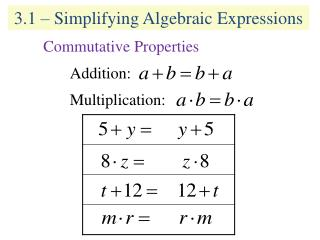DownloadDownload Presentation3.1 – Simplifying Algebraic Expressions

# 3.1 – Simplifying Algebraic Expressions

Download Presentation## 3.1 – Simplifying Algebraic Expressions

- - - - - - - - - - - - - - - - - - - - - - - - - - - E N D - - - - - - - - - - - - - - - - - - - - - - - - - - -
##### Presentation Transcript

1. 3.1 – Simplifying Algebraic Expressions Commutative Properties Addition: Multiplication:

2. 3.1 – Simplifying Algebraic Expressions Associative Properties Addition: Multiplication:

3. 3.1 – Simplifying Algebraic Expressions Distributive Property of Multiplication

4. 3.1 – Simplifying Algebraic Expressions Definitions Term: a number or a product of a number and variables raised to a power. Coefficient: the numerical factor of each term. Constant: the term without a variable. Like Terms: terms with the same variable factors, but may have different coefficients.

5. 3.1 – Simplifying Algebraic Expressions Simplify each expression by combining like terms.

6. 3.1 – Simplifying Algebraic Expressions Simplify each of the expressions by using the distributive property. Simplify each of the expressions.

7. 3.1 – Simplifying Algebraic Expressions Simplify each of the expressions.

8. 3.1 – Simplifying Algebraic Expressions Find the perimeter of the square. 2x cm. Find the area of the rectangular lawn. 3 yds. (12y + 9) yds.

9. 3.2 – Solving Equations Addition and Multiplication Properties

10. 3.2 – Solving Equations Addition and Multiplication Properties

11. 3.2 – Solving Equations Addition and Multiplication Properties

12. 3.2 – Solving Equations General Review Translate each phrase into an algebraic expression. Use “x” as the number. The sum of negative three an a number. -3 + x Negative five decreased by a number. -5 - x A number subtracted from eighty-three. 83 - x The quotient of a number and -4.

13. 3.2 – Solving Equations General Review Translate each phrase into an algebraic expression. Use “x” as the number. The product of 5 and a number, decreased by 25. 5x - 25 Twice the sum of a number and 3. 2(x + 3) The quotient of 29 and twice a number.